# RF Tutorial Lesson 14: Analyzing a Communications System Using Virtual Blocks

 Tutorial Project: Analyzing a Communications System Using Virtual Blocks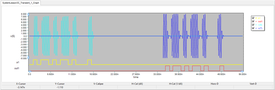Objective: In this project, you will build a QAM modulator and demodulator pair using virtual blocks and will use them to simulate a communication link. Concepts/Features: Communication Link Modulator Demodulator Communication Channel Binary Dara Carrier Frequency QAM Modulation Minimum Version Required: All versions

## What You Will Learn

In this tutorial you will use RF.Spice's black-box virtual blocks to model a Quadrature Amplitude Modulation (QAM) communication system and construct a pair of transmitter and receiver circuits. Then you will use a long lossy transmission line as the channel to connect the transmitter and receiver circuits. You will simulate the transmission of a binary data packet through this communication link.

## Overview of the COMM System Architecture

Quadrature Amplitude Modulation (QAM) is a digital modulation scheme that is similar in nature to the analog AM. A binary data sequence is converted to a pulse train that serves as the baseband signal of the communications system. The baseband signal is split into two equal parts and the two parts are mixed with synchronous cosine and sine versions of the carrier frequency fc. The up-converted signals are then summed together with opposite signs and the resulting QAM signal is sent to the channel. The figure below shows the basic simplified architecture of the QAM communications system. There can be additional blocks including gain stages and signal shaping filters, which have been omitted here for the sake of simplicity.

On the receiver side, the received QAM signal from the channel is split into two equal parts and the two parts are mixed with synchronous cosine and sine versions of the carrier frequency fc. The down-converted signals are then summed together with opposite signs and the resulting signal goes through a peak detector. The output analog signal then passes through a comparator to recover the transmitted binary sequence.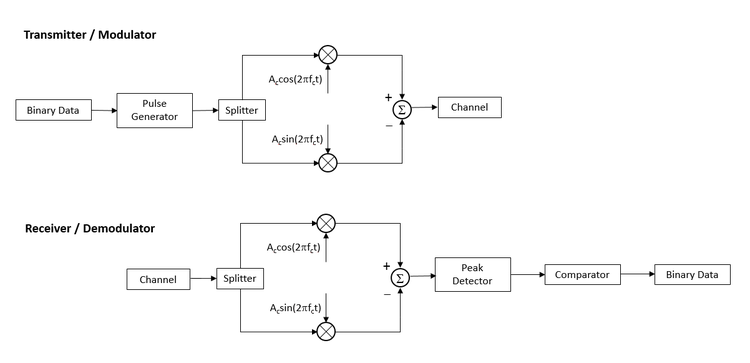The block diagram of a QAM communications system.

## Building and Testing the Modulator Circuit

The following is a list of parts needed for this part of the tutorial lesson:

Part Name Part Type Part Value
A1 Digital Source Data TBD
A2 1-Bit DAC Conversion Bridge Defaults, out_high = 5, t_rise = 100p, t_fall = 100p
X1 Wilkinson Power Divider Defaults, fc = 1GHz
X2 QAM Modulator Block Defaults, fc = 3GHz, ac = 5
R1 - R2 Resistor 50

To generate a binary sequence for this project, , you will use a "Digital Source" followed by a DAC conversion bridge. The data rate will be 1GHz or 1G sample/s. In other words, we assume a clock rate of 1GHz with a period of 1ns and a pulse with of 500ps, The input binary sequence is:

0101 1010 1101 0001 0000

The digital source device itself doesn't have an explicit clock input. You set the timing through its Time-Value Array table as shown in the table below:

Time State
0 0
1ns 1
2ns 0
3ns 1
5ns 0
6ns 1
7ns 0
8ns 1
10ns 0
11ns 1
12ns 0
15ns 1
16ns 0
20ns 0

Place and connect all the parts as shown in the figure. The DAC conversion bridge generates a pulse train of binary voltage levels 0V and +5V based on the input bit sequence. For the QAM modulator, you will use a 3GHz carrier frequency with a carrier amplitude of 5V. For the signal splitter, you will use the Wilkinson power divider you explored earlier in RF Tutorial Lesson 12. Set the center frequency of the Wilkinson power divider to fc = 3GHz and set its power ratio to K =1 for equal split between the two output ports.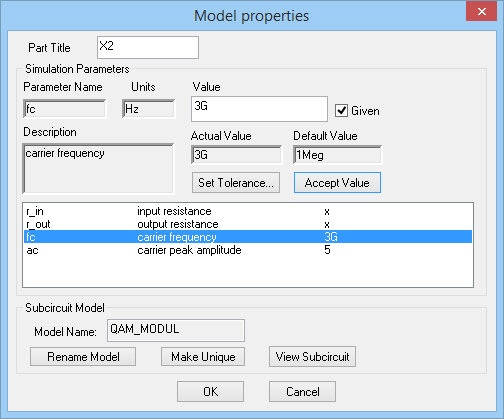The property dialog of the QAM Modulator block.

Run a Transient Test of your modulator circuit with the following parameters:

 Start Time 0 25n 10p 10p a1(Digital), v(6)

The results are shown in the figure below. Note that a very small time step ceiling make result in long computation time. Here we have chosen a Step Ceiling of 10ps to speed up the simulation.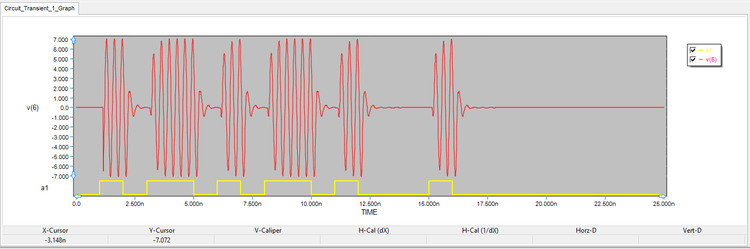The graph of the digital input binary sequence and the analog output voltage of the QAM modulator circuit.

## Building and Testing the Demodulator Circuit

The following is a list of parts needed for this part of the tutorial lesson:

Part Name Part Type Part Value
VREF DC Voltage Source 4V
X1 AM Source VA = 15V, fc = 3G, MDI = 1, fs = 0.3G
X2 Resistive Power Divider Defaults, len_in = 2mm, len_out = 2mm
X3 QAM Modulator Block Defaults, fc = 3GHz, ac = 5V
A1 Limiter Block Defaults, gain = 5
X4 Ideal Full-Wave Rectifier Block Defaults
X5 Ideal Comparator Block Defaults, Gain
R1 Resistor 10
R2 Resistor 100
C1 Capacitor 100p
A2 1-Bit ADC Conversion Bridge Defaults, in_high = 2, t_rise = 100p, t_fall = 100p
Out1 Digital Output N/A

In this part of the tutorial lesson, you will build a QAM demodulator circuit. To test your demodulator circuit, you will initially use an AM-modulated voltage source for the input signal. This source is accessible from Menu > Parts > Waveform Generation Blocks > Modulated Waveforms > Single-Tone AM Modulated Source. It represents a sinusoidal baseband signal of frequency fs modulated on a carrier frequency of fc. The AM waveform can be expressed as:

$y(t) = A_c \left( 1 + m x(t) \right) cos(2\pi f_c t) = A_c \left( 1 + m A_s cos(2\pi f_s t) \right) cos(2\pi f_c t)$

where fc and fs are the carrier and signal frequencies, respectively, and m (the MDI parameter) is the modulation index or depth. m = 0 means no modulation, and m = 1 represent full modulation. You are going to feed an AM modulated signal with a signal frequency of 300MHz, a carrier frequency of 3GHz, a carrier amplitude of 15V and a modulation index of m = 1.Most RF devices in RF.Spice A/D have a center frequency fc parameter that must be specified in GHz. On the other hand, the frequency of analog sources and most virtual blocks has to be specified in Hz.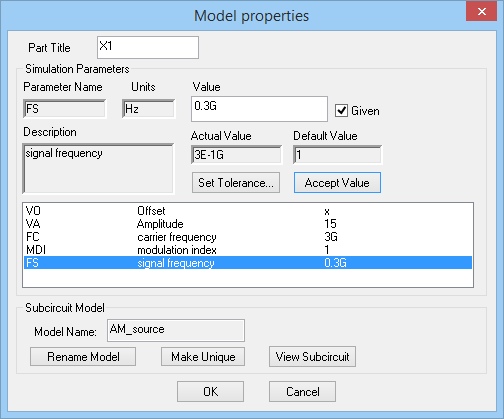The property dialog of the AM-Modulated Source.

Place and connect all the parts as shown in the figure below. For the receiver circuit, instead of a Wilkinson power divider, you will use a simpler resistive power divider. Set the lengths of the input and output feed lines to a minimum: len_in = len_out = 2mm. For this part, you will use the "Limiter Block" simply as an amplifier with a gain of 5. For the peak detector block of the receiver circuit, you will use a full-wave bridge rectifier together with a simple RC filter. The analog binary output of this circuit fluctuates between low and high voltage levels. Finally, the Ideal Comparator Block compares the output of the peak detector circuit to a fixed DC voltage of +4V. Set the gain of the comparator to 10. This may also serve as an IF or baseband amplifier. The output of the comparator is converted to a digital output using s ADC bridge.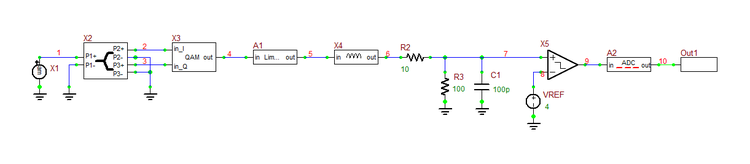The schematic of the QAM Demodulator circuit.

Run a Transient Test of your modulator circuit with the following parameters:

 Start Time 0 25n 10p 10p v(1), v(4), v(7), out1(digital)

The results are shown in the figure below. As you can see from the figure, the voltage signal v(7) at the output of the peak detector (plotted in dark blue) is a good reconstructed replica of the baseband 300MHz sinusoidal signal. Compare this signal to the envelope of the input AM-modulated signal plotted in yellow. You can use the "Delta Line Mode" of the graph window to measure the period or clock rate of the binary output, which is about 3.34ns consistent with the signal frequency of fs = 300MHz.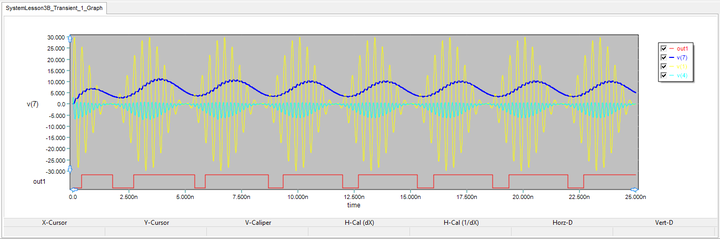The graph of the analog input voltage (yellow), output of the QAM block v(4), output of the peak detector v(7) and the digital output of the QAM Demodulator circuit.

## Putting the Transmitter and Receiver Together With the Channel

The following is a list of parts needed for this part of the tutorial lesson:

Part Name Part Type Part Value
A1 Digital Source Data TBD
A2 1-Bit DAC Conversion Bridge Defaults, out_high = 5, t_rise = 100p, t_fall = 100p
X1 Wilkinson Power Divider Defaults, fc = 1GHz
X2, X5 QAM Modulator Block Defaults, fc = 3GHz, ac = 5V
XTL1 Generic T-Line Defaults: Z0 = 50, eeff = 1, alpha = 0.5dB/m, len = 10,000mm
R1 - R2 Resistor 50
VREF DC Voltage Source 2V
X3 Ideal Buffer Block Defaults
X4 Resistive Power Divider Defaults, len_in = 2mm, len_out = 2mm
A1 Limiter Block Defaults, gain = 5, out_lower_limit = -1V, out_upper_limit = 1V
X6 Ideal Full-Wave Rectifier Block Defaults, gain = 5
X7 Ideal Comparator Block Defaults, gain = 10
R1 Resistor 50
R2 Resistor 60
R2 Resistor 80
C1 Capacitor 10p
A4 1-Bit ADC Conversion Bridge Defaults, in_high = 2, t_rise = 100p, t_fall = 100p
Out1 Digital Output N/A

In this part of the tutorial lesson, you will put the QAM modulator and demodulator circuits of the previous parts together with a channel to simulate a communication link. In a wireless system, the channel is the free space between the transmit and receive antennas. One way of modeling the free-space channel in RF.Spice A/D is to use a dependent voltage source at the input of the receiver that is driven by the voltage at the output of the transmitter. The proportionality constant can be set to a very small number representing the free-space path loss. For this project, however, you will use a wired channel or cable represented by a lossy transmission line segment. In that case, the receiver can be directly connected to the output port of the T-Line segment. You will use a TEM line with Z0 = 50Ω, eeff = 1 and alpha = 0.5dB/m. At a length of 10m, the total single attenuation due to the channel will be 5dB.

You are now ready to put it all together. This time you will send the QAM-modulated output of the transmitter circuit you built earlier through the transmission line channel and will feed it as the input of your receiver circuit. This signal will replace the AM-modulated voltage source you used in the previous section to feed your QAM demodulator circuit. For this part of the project, you will place an Ideal Buffer Block for isolation between the transmission line and the rest of the receiver circuit. The Limiter Block will both amplify and clip the signal from the top and bottom. Set the gain to 5 and set the lower and upper limits of the output voltage to -1V and +1V, respectively. Also define a gain of 5 for the Ideal Full-Wave Rectifier Block.

Place and connect all the parts as shown in the figure below. Note that the part values of the peak detector's lowpass filter have changed from the previous part. Also, the reference voltage of the Ideal Comparator Block has been decreased to 2V.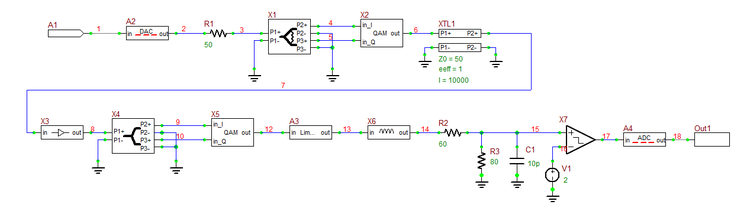The schematic of the COMM link including the transmitter and receiver circuits and the cable channel.

As the transmitted signal propagates through the transmission line, besides phase change or time delay, it also gets attenuated by 5dB over its whole length. The QAM-modulated signal signal arrives at the input of the receiver after a time delay equal to:

$\Delta t = \frac{L}{c} = \frac{10m}{3\times 10^8 m/s} = 33.3ns$

To plan your Transient Test, keep in mind that the input binary sequence has a total duration of 20ns, of which the last 4ns are zeros (silent). In addition, the channel causes a time delay of 33.3ns. So choose the following parameters to run a Transient Test of your QAM data communications link:

 Start Time 0 55n 10p 10p a1(digital), v(6), v(7), out1(digital)

The results are shown in the figure below. The plots in light and dark blue represent the voltage signals at the input and output of the long lossy transmission line. As you can from the figure, the demodulated signal at the digital output represents a fairly good replica of the input binary sequence.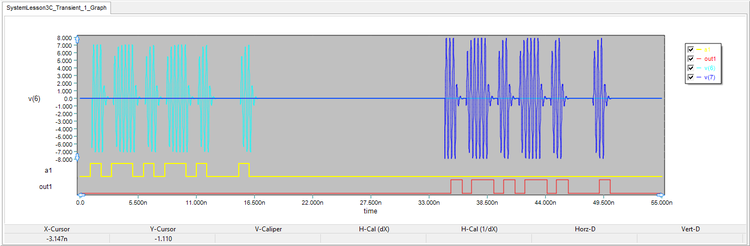The graph of the digital input and output binary sequences of the QAM Modulator-Demodulator combo circuit, plotted together with the voltages at the input v(6) and output v(7) of the transmission line channel.